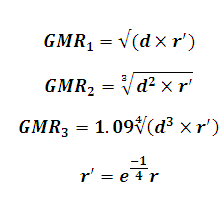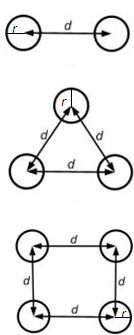# GMR(Geometric Mean Radius) of Bundled Conductors for basic configurations Calculator

This Calctown Calculator calculates the Geometric Mean Radius of bundled conductors of the given basic configurations.

Please enter the type of configuration whose GMR is to be calculated.

m
m

#### Result

m
m
mClick here to view image

where

GMR = Geometric Mean Radius

d = distance between strands

r = radius of individual lines